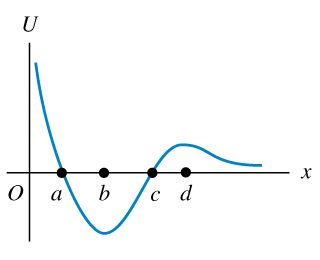# Problem: A marble moves along the x-axis. The potential-energy function is shown in the figure .At which of the labeled x-coordinates is the force on the marble zero?Which of the labeled x-coordinates is a position of stable equilibrium?Which of the labeled x-coordinates is a position of unstable equilibrium?

###### FREE Expert Solution
95% (278 ratings)
###### Problem Details

A marble moves along the x-axis. The potential-energy function is shown in the figure.

At which of the labeled x-coordinates is the force on the marble zero?

Which of the labeled x-coordinates is a position of stable equilibrium?

Which of the labeled x-coordinates is a position of unstable equilibrium?

Frequently Asked Questions

What scientific concept do you need to know in order to solve this problem?

Our tutors have indicated that to solve this problem you will need to apply the Force & Potential Energy concept. You can view video lessons to learn Force & Potential Energy. Or if you need more Force & Potential Energy practice, you can also practice Force & Potential Energy practice problems.